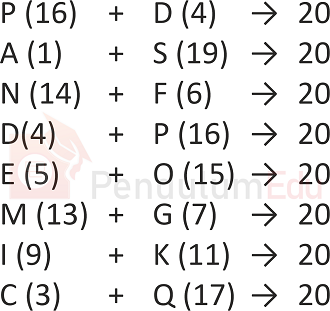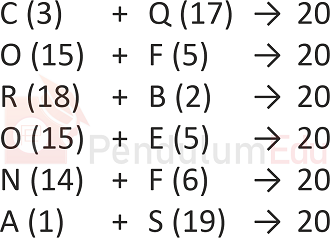Question of The Day10-12-2020

If  "PANDEMIC" is coded as "DSFPOGKQ", then what will be the code for "CORONA"?

Correct Answer : c ) QEBEFS

Explanation :

Let us assume A is numbered as 1, B as 2 and so on till Z being numbered as 26.

According to the question,

PANDEMIC is coded as DSFPOGKQ

Here, the common logic followed is that the sum of the numerical value of the corresponding alphabets in the word and code is 20.

Depicting the logic, we getSimilarly, code for CORONA will beThus, CORONA will be coded as QEBEFS

Hence, (c) is the correct answer.

Such type of question is asked in various government exams like SSC CGL, SSC MTS, SSC CPO, SSC CHSL, RRB JE, RRB NTPC, RRB GROUP D etc.

Read Daily Current Affairs, Banking Awareness, Hindi Current Affairs, Word of the Day, and attempt free mock tests at PendulumEdu and boost your preparation for the actual exam.0